Next: 4.4 Boolean Operations Up: 4.3 Sparse Field Implementation Previous: 4.3.2 Pruning and Consistency

## 4.3.3 Dilation

The set of defined grid points in the H-RLE data structure is as a result of the pruning procedure a subset of. The LS values are all in the range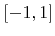due to restriction (4.8). For the next time step the LS values of all new active grid points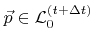must be known. Furthermore, the LS values of neighboring layers, as needed for the time integration scheme, must be updated. This requires a dilation procedure which extends the set of defined grid points and calculates their corresponding LS values using the update scheme (3.29).

To extend the set of defined grid points on the positive and the negative side by a single layer, a stencil of iterators, which allows access to neighbor points, is moved over the H-RLE data structure. If any iterator from the stencil encounters a defined grid point, a defined grid point is inserted into the new H-RLE data structure. If the position of the central iterator represents a defined grid point, its LS value is simply copied. Otherwise, the LS value must be determined using the update scheme (3.29) and the LS values of neighbor points, which can be accessed by the non-central iterators. Here, positive and negative undefined runs are regarded to have LS values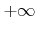or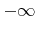, respectively. Since at least one iterator is at the position of a defined grid point the LS value of the newly inserted grid point is always defined. After completing the iteration, the old H-RLE data structure can be deleted. The new H-RLE data structure now contains all previously defined grid points along with the newly added defined grid points.

After the first application of the dilation procedure, the LS values of all grid points in layers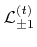are updated. Since the CFL condition (3.31) implies that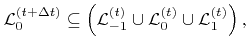(4.9)

the updated LS values are available for all new active grid points. Similarly, a second run of the dilation procedure which updates all points in layers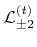ensures that at least for all grid points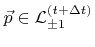the updated LS values are available, and so on. The number of repetitions of the dilation procedure depends on how many additional layers of defined grid points are needed for the time integration scheme. As an example, for the second order approximation, which requires two additional layers, three iterations are necessary in total.

However, this procedure also adds defined grid points to the H-RLE data structure, which are not really needed for the finite difference scheme. If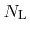additional layers are necessary, the last iteration which updates all points of layers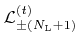also adds some new points which are actually members of. The insertion of these grid points can easily be avoided according to (3.26), if only grid points for which the new LS value fulfillsare added. The procedure can be further optimized by inserting new grid points as late as possible to speed up successive iterations over the H-RLE data structure. It is favorable to add only defined grid points with an absolute LS value not larger than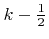during the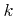-th iteration.

The dilation procedure scales with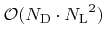, where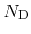is the number of defined grid points before dilation andis the number of added layers. Hence, this algorithm is only efficient for small, which is the case, if first (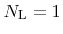) or second order finite difference schemes () are used. For larger, an algorithm similar to the fast marching method  could probably be the better choice. However, this technique would require random access to the H-RLE data structure resulting in a non-linear performance with respect to.

The pruning and consistency check, as described in the previous section, can be included during the first dilation cycle. This avoids an iteration over the H-RLE data structure and accelerates the sparse field method.Next: 4.4 Boolean Operations Up: 4.3 Sparse Field Implementation Previous: 4.3.2 Pruning and Consistency

Otmar Ertl: Numerical Methods for Topography Simulation